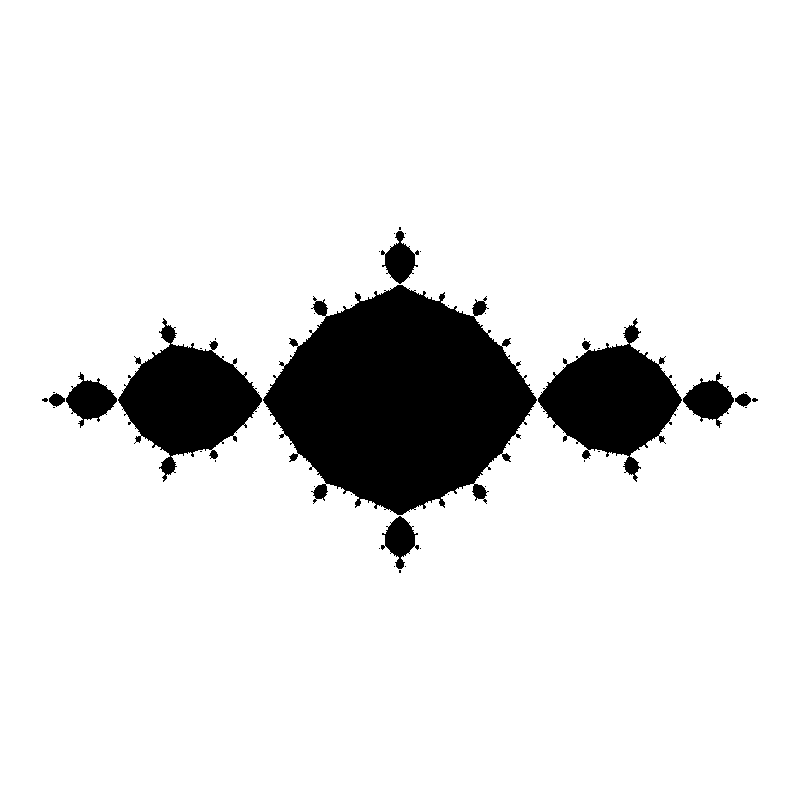A document from MCS 275 Spring 2022, instructor David Dumas. You can also get the notebook file.

# Filled Julia sets: Comparing methods¶

MCS 275 Spring 2022 - David Dumas

## Convention¶

We'll compute the filled Julia set of $f(z) = z^2 + c$. Previously, we only discussed $c=0$ and $c=-1$ in class, and other values of $c$ were added to the example notebook between lectures.

This notebook shows three ways to do the same thing, and all are set to make a 800x800 image with 500 iterations. The first method (using Python for loops) is very slow!

## Setup¶

In :
import numpy as np
from PIL import Image
import time

In :
# Some values of c to try

c_circle = 0
c_basilica = -1
c_rabbit = -0.123 + 0.745j
c_curly_snowflake = -0.4-0.582j  # my name for this!
c_airplane = -1.75487766624669276


## Using Python for loops¶

In :
def escapes(c,a,n):
"""Determines if orbit of a under z->z^2+c escapes |z|<2 within
n iterations.
"""
for _ in range(n):
if abs(a) >= 2:
return True
a = a*a+c
return False

def filled_julia_set_for(c,xmin=-1.8,xmax=1.8,ymin=-1.8,ymax=1.8,xres=500,yres=500,maxiter=100):
"""Create PIL image of the filled Julia set of z^2+c"""
x = np.linspace(xmin,xmax,xres)
y = np.linspace(ymin,ymax,yres)

xx, yy = np.meshgrid(x,y)
zz = xx + 1j*yy

f = lambda z: escapes(c,z,maxiter)

res = np.zeros_like(zz,dtype="bool")
for i in range(len(x)):
for j in range(len(y)):
res[j,i] = f(zz[j,i])  # j is the row, and indexing is row,column

return Image.fromarray( 255*res.astype("uint8"), mode="L" ).transpose(method=Image.FLIP_TOP_BOTTOM)

In :
t_start = time.time()
img = filled_julia_set_for(c_basilica,maxiter=500,xres=800,yres=800)
t_end = time.time()

print(t_end-t_start,"seconds")
img

12.515106916427612 seconds

Out:## Using numpy.vectorize¶

This is the method we discussed in Lecture 27, but the code has been cleaned up a bit.

In :
def escapes(c,a,n):
"""Determines if orbit of a under z->z^2+c escapes |z|<2 within
n iterations.
"""
for _ in range(n):
if abs(a) >= 2:
return True
a = a*a+c
return False

def filled_julia_set_vectorize(c,xmin=-1.8,xmax=1.8,ymin=-1.8,ymax=1.8,xres=500,yres=500,maxiter=100):
"""Create PIL image of the filled Julia set of z^2+c"""
x = np.linspace(xmin,xmax,xres)
y = np.linspace(ymin,ymax,yres)

xx, yy = np.meshgrid(x,y)
zz = xx + 1j*yy

f = lambda z: escapes(c,z,maxiter)

return Image.fromarray( 255*np.vectorize(f)(zz).astype("uint8"), mode="L" ).transpose(method=Image.FLIP_TOP_BOTTOM)

In :
t_start = time.time()
img = filled_julia_set_vectorize(c_basilica,maxiter=500,xres=800,yres=800)
t_end = time.time()

print(t_end-t_start,"seconds")
img

3.8326313495635986 seconds

Out:## Using numpy ufuncs¶

An even faster approach is to use numpy's overloading of the arithmetic operations to apply $z^2+c$ to each element of the grid simultaneously.

If we do this naively, applying the function maxiter times to every point, many of the calculations will overflow. To prevent this, we can use a mask (boolean array) to stop applying the function as soon as a point leaves $|z|<2$.

In [ ]:
zz = zz*2-1

In :
def filled_julia_set_ufunc(c,xmin=-1.8,xmax=1.8,ymin=-1.8,ymax=1.8,xres=500,yres=500,maxiter=100):
"""Create PIL image of the filled Julia set of z^2+c"""
x = np.linspace(xmin,xmax,xres)
y = np.linspace(ymin,ymax,yres)

xx, yy = np.meshgrid(x,y)
zz = xx + 1j*yy

m = np.ones_like(zz,dtype="bool") # Mask: True means we are
# still applying f to that
# point in the grid.

for _ in range(maxiter):
zz[m] = zz[m]**2 + c # apply z^2+c to each point where m is True
m[m] &= np.abs(zz[m])<2 # Set mask to False for any point
# that now has abs(z)>=2

return Image.fromarray( 255*((np.abs(zz)>=2).astype("uint8")), mode="L" ).transpose(method=Image.FLIP_TOP_BOTTOM)

In :
t_start = time.time()
img = filled_julia_set_ufunc(c_basilica,maxiter=500,xres=800,yres=800)
t_end = time.time()

print(t_end-t_start,"seconds")
img

0.6942436695098877 seconds

Out:In [ ]: